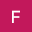# Math Solver

3.3
6.4K reviews
500K+Everyone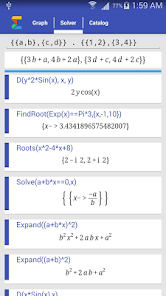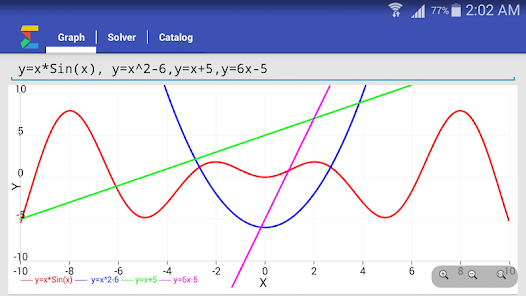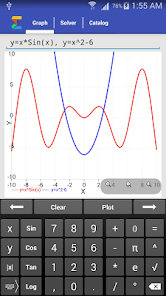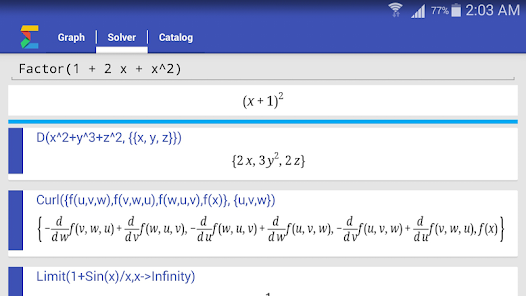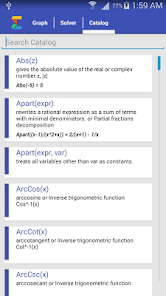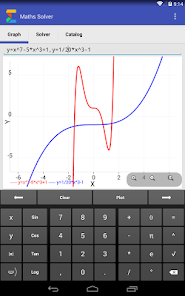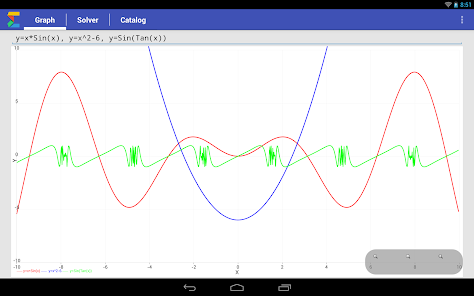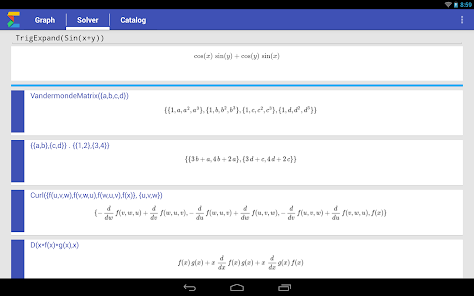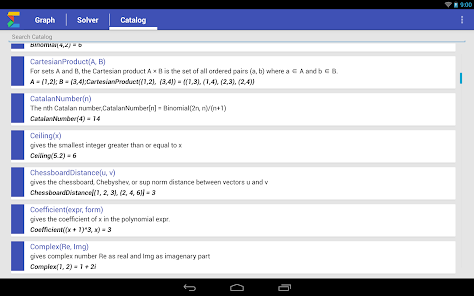Solve Math problems and Plot functions.
Math Solver is a full featured scientific calculator and graphing calculator with widest range of functions available.
Solves problems in Basic Math, Algebra, Complex Numbers, Trigonometry, Integral and Differential Calculus, Set Theory, Matrix and vector Algebra

Solve equations and system of equations
Find derivatives, definite and indefinite integral
Support for trigonometric inverse-trigonometric and hyperbolic functions

No network access required! Works offline
It has a offline Catalog with details of each function with example.

* Enter multiple comma separated functions to plot them.
* Press back button to make graph full screen by hiding keyboard
* Press on any example in Catalog to copy it to Solver tab
* Trigonometric functions default input is in radian i.e to calculate Sin(90Degree) to calculate Sine of 90 degree.

Note- 1:- It doesn't solve word problems.
Note- 2:- It doesn't show steps to solve a problem. It solve shows final answer.
Updated on
Jun 4, 2017

## Data safetyNo information available
3.3
6.4K reviews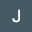Jeylyn Muchena
July 27, 2020
It does not show the working
57 people found this review helpful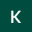Kelvin Aidoo
May 16, 2022
Better in terms of mathematics